Get instant live expert help with Excel or Google Sheets“My Excelchat expert helped me in less than 20 minutes, saving me what would have been 5 hours of work!”

#### Post your problem and you’ll get Expert help in seconds.

Your message must be at least 40 characters
Our professional Expert are available now. Your privacy is guaranteed.

# Count specific words in a range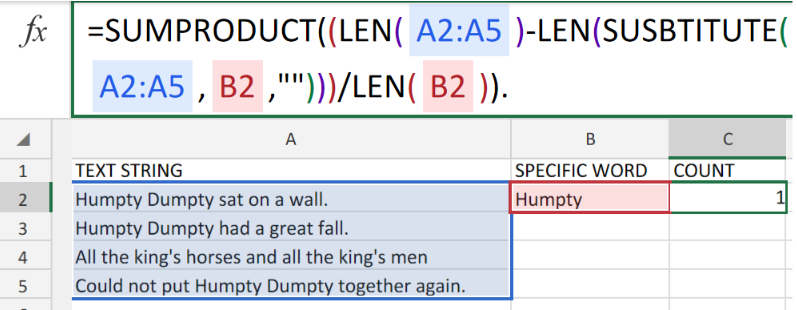Figure 1. of Count Specific Words in a Range.

In order for us to determine the number of times a specific word/text (or a substring) is occurring within a range of cells, we are going to utilize SUMPRODUCT, SUBSTITUTE and LEN Functions. This tutorial will step through the process.

## Generic Formula

`=SUMPRODUCT((LEN(rng)-LEN(SUBSTITUTE(rng,txt,"")))/LEN(txt))`

## How to use the SUMPRODUCT, SUBSTITUTE and LEN Functions in Excel

In the generic formula syntax stated above, the text range to check is represented by “rng”, while “text” is representing the specific word being checked for.

We can achieve this by following three simple steps;

1. Collect and arrange our data values within our worksheet. Label the columns appropriately. See example illustrated below.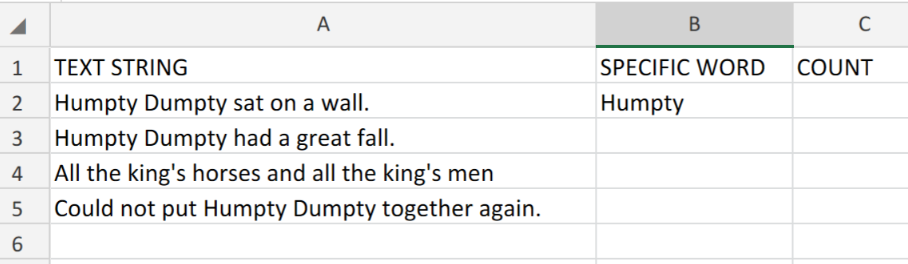Figure 2. of Text Data for Checking in Excel.

1. Our purpose here is to determine the number of times that the word “Humpty” is occurring within the text strings in column A of our worksheet.

The formula syntax which we will enter into the formula bar for cell C2 to determine the count is;

`=SUMPRODUCT((LEN(A2:A5)-LEN(SUBSTITUTE(A2:A5,B2,"")))/LEN(B2))`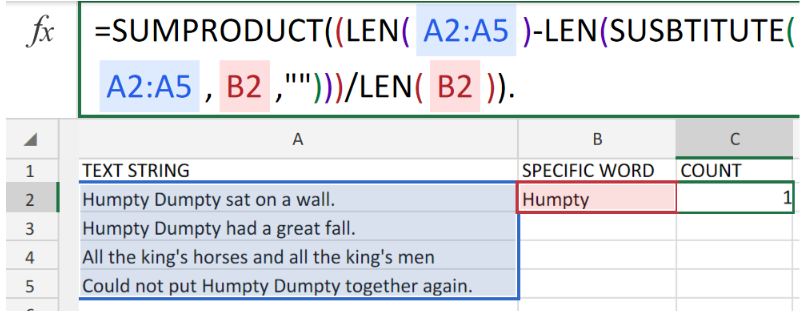Figure 3. of SUMPRODUCT, SUBSTITUTE and LEN Functions in Excel.

Excel checks our text string and returns the specified word count in column C of our worksheet.

1. Modify and copy the formula syntax into the cells down the column to achieve the desired results.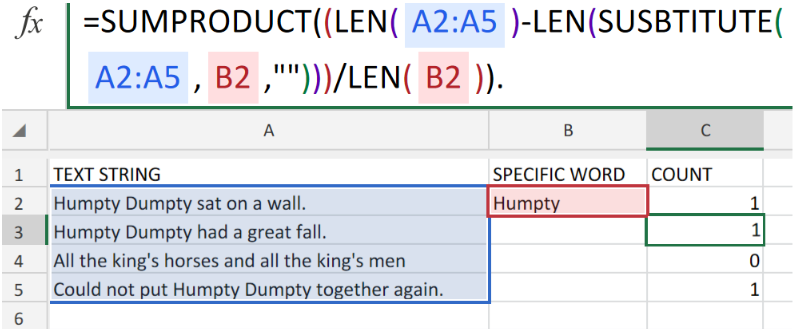Figure 4. of SUMPRODUCT, SUBSTITUTE and LEN Functions in Excel.

## Note

• Our rng in the example illustrated above is A2:A5
• Our text is in the example illustrated above is “Humpty”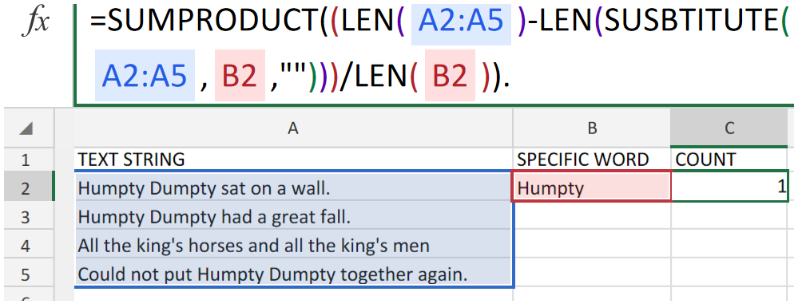Figure 5. of Final Result.

Instant Connection to an Expert through our Excelchat Service:

Our live Excelchat Service is here for you. We have Excel Experts available 24/7 to answer any Excel questions you may have. Guaranteed connection within 30 seconds and a customized solution for you within 20 minutes.

### Did this post not answer your question? Get a solution from connecting with the expert.Another blog reader asked this question today on Excelchat:## Subscribe to Excelchat.coAnother blog reader asked this question today on Excelchat: